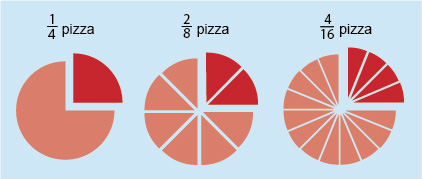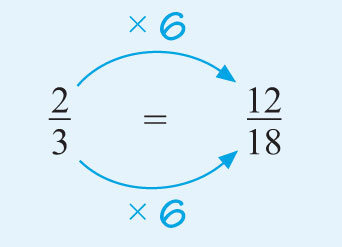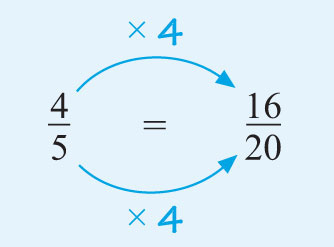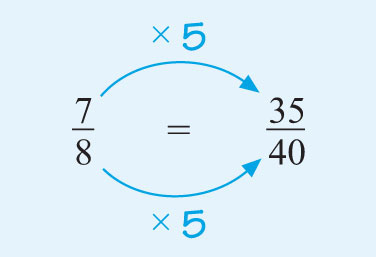Succeed with maths – Part 1

Start this free course now. Just create an account and sign in. Enrol and complete the course for a free statement of participation or digital badge if available.

Free course

2.1 Working with equivalent fractions

Here’s a slightly different way to help you visualise this process by imagining these circles as pizzas. You can see that of a pizza is the same as of a pizza, and also the same as of a pizza. So all these fractions are equivalent and can be simplified to . This is similar to our example of folding a piece of paper.Figure 7 Pizza maths

Below are some examples for you to try but before you do that you might like to view this video on equivalent fractions.

Skip transcript

Transcript

Narrator
So we've got this fraction written here, 2/3. What I want you to do is pause this video and try to think of any other fractions that are the same that are equivalent to this fraction right over here that essentially represent the same number. So to do that, let's visualize what 2/3 is. So let me draw a whole here. Let me draw a whole, and I'm going to divide it into three equal sections. So that is my whole.I'm drawing three equal sections I'm going to try to draw and make them as equal as i--I can do a little better job than that. So that's 1, 2, 3. Three equal sections. And so 2/3 would represent two out of those three equal sections. So actually I can spray paint this. So that's 1/3 right over here, and then this is 2/3. So we have two of the three equal sections. So that is 2/3. And now let me copy and paste that so we can think about other ways to represent this fraction. So copy. And then let me paste t.
I'll do it once, and then I'll do it another time. And I could do this multiple times, but I'll do it two other times right over here. So there's a couple of things we could do. The first option is we could take this and we could draw a horizontal line that divides each of these three sections into two sections. So let's do that.
So now, how many equal sections do I have? I have 1, 2, 3, 4, 5, 6 equals sections. And how many of those equal sections are actually shaded in now. Well, we see it's 1, 2, 3, 4. 4/6. So notice, 4/6 is the exact same fraction of the whole as 2/3. These are equivalent fractions. We could say that 2/3 is equal to 4/6. Now, we could do something very similar. Instead of dividing each of these thirds into two, we could divide each of these thirds into three. So I could draw three horizontal lines here. So let's see 1, 2, 3. So now I have divided what was in three equal sections, I now have three times as many sections. I have 1, 2,, 3, 4, 5, 6, 7, 8, 9 equals sections. And then which of those are actually shaded in? We have 1, 2, 3, 4, 5, 6. 6. So 2/3, which is equal to 4/6, is also equal to 6/9. All three of these are equivalent fractions, 2/3, 4/6, and 6/9. And if you were to put them on the number line, the same thing would happen. So let's do that. Let's draw a number line here. Let's say that's 0.
And I'm just going to focus on between 0 and 1. And obviously we can go beyond that. And let's divide it first into thirds. So this is 1/3 and 2/3. So we already know this would represent 1/3 and this is 2/3. We've gone two of the equal spaces of the three on the way to 1. We've divided the section between 0 and 1 into three equal spaces. Now, what for 4/6 be? Well, now we would just have to divide this into 6 equal spaces. So 1, 2, 4, 4, 5, 6. And 4/6, that would be 4 out of the 6 spaces on the way to 1. So 1, 2, 3, 4. So this number is also equal to 4/6. And you could do the same thing if you want to think about ninths.
So what we could do, we could put 1, 2, 3, 4, 5, 6, 7, 8, 9. Now I've split this part of our number line between 0 and 1 into 9 equal spaces. Well, what would 6/9 be? Well, 1, 2, 3, 4, 5, 6. Once again, the exact same point on the number line. It's an equivalent fraction. 6/9 is equal to 2/3 is equal to 4/6.
End transcript

Interactive feature not available in single page view (see it in standard view).

Remember – when carrying out the activity there may be more than one way to arrive at the answer, and you must divide or multiply both the numerator and the denominator by the same number at each step.

Activity 2 Equivalent fractions

Timing: Allow approximately 10 minutes

(a) Use your knowledge of equivalent fractions to determine the missing numbers. Remember that both the numerator and the denominator must be multiplied or divided by the same number.

(i)

Comment

The denominator of the fraction on the left is 3. What do you have to multiply it by to get 18? Remember that if you multiply the denominator by a particular number, you must do the same to the numerator to keep the fractions equivalent.

(a) (i) The 3 is multiplied by 6 to reach 18, so this is the number that is used to multiply both the numerator and the denominator. The missing number is 12.Figure 8

(ii)

(a) (ii) Similarly, since 5 × 4 = 20, multiply the numerator and the denominator by four. The missing number is 16.Figure 9

(b) Which of the following three fractions are equivalent to each other?

(i)

(ii)

(iii)

(b) Multiplying the numerator and the denominator of the first fraction by five gives:Figure 10

Only the two fractions labelled (i) and (ii) are equivalent.

You cannot create from by multiplying the numerator and the denominator by the same number.

If you multiply by 7 you get , which isn’t . If you multiply by 8 you get , which isn’t , either.

(c) Simplify the following fractions:

(i)

(ii)

(iii)

(iv)

Comment

What number can divide exactly into both the numerator and the denominator of each fraction? Remember, there could be more than one option or step to fully simplify these.

(c)

(i) Dividing the top and the bottom by 2 gives .

(ii) Dividing the top and the bottom by 10 (or by 5 and then by 2) gives .

(iii) Dividing the top and the bottom by 9 and then by 3 gives .

(iv) Dividing the top and the bottom by 4 and then 3 gives .

(Of course it does not matter which number you divide by first, and there are even more choices than the ones shown.)

If you find it difficult to spot the numbers to divide by, try to work systematically by trying 2, 3, 5 … in turn.

In the next section, you can try an activity where you need to write your own fractions from information you are given. Remember to show these in their simplest form – this is the way that fractions should be shown.